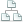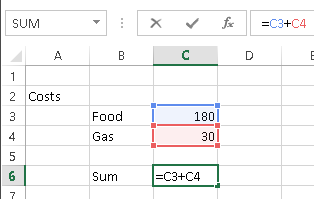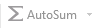Home >Tips >Excel Formulas, 1Site map

# Use Formulas in Excel – sums

## An Excel tutorial by Peter KalmstromIn the demo below Peter Kalmstrom goes through the basics of Excel formulas. The possibility to tell Excel to calculate is a crucial feature and the most important reason to use it, and here Peter explains how to tell the program what to count on = how to create a formula.

When you use a formula Excel calculates with the values of the cells you have given in thet formula and gives the result in a separate cell. When the values in the cells are changed the figure in the result cell will change automatically.Excel is used for all kinds of calculations – from personal budgets to very advanced prognoses – but the calculations cannot be accurate if the formulas are not correct. With this demo Peter wants to set Excel new beginners on the right track.

Peter shows:

• What kind of information the cells in an Excel spreadsheet can contain and how you can see if Excel treats data as text or numbers.
• How you should NOT use Excel for calculations
• How to calculate a sum using a formula with the plus sign
• How to calculate a difference using a formula with the minus sign
• The functions of "sum", parenthesis and colon in formulas
•How to use the AutoSum button
Peter uses Excel 2013 for his demo, but the formulas are the same for earlier versions of Excel.